### Home > A2C > Chapter Ch11 > Lesson 11.2.4 > Problem11-94

11-94.
1. For an object shot into the air, its height h in feet above the ground after t seconds is given by the equation h = 80t − 16t2. Use this equation to answer the following questions. Homework Help ✎

1. For what times is the object on the ground?

2. For what domain is this function reasonable?

3. How long did it take the object to hit the ground?

4. For what times is the height greater than 64 feet?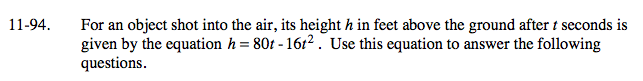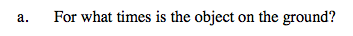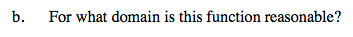Substitute 0 for h.

80t − 16t2 = 0

It is on the ground at 0 and 5 seconds.

Factor the equation.

16t(5 − t) = 0

When is the height positive?

D: 0 ≤ t ≤ 5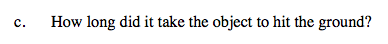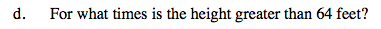At what time will the object be on the ground, not including when it starts?

5 seconds

16t 2 − 80t + 64 = 0

Substitute 64 for h and solve for t.

Factor the equation.

(t − 1)(t − 4)

Simplify.

t 2 − 5t + 4

t = 1, 4

Solve for t.

Use these values to find height greater that 64 ft.

1< t < 4